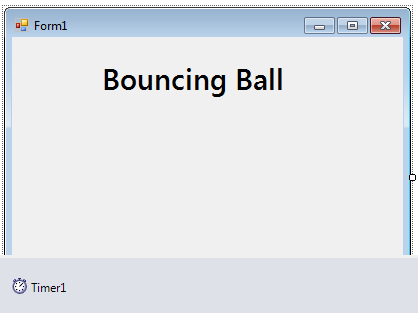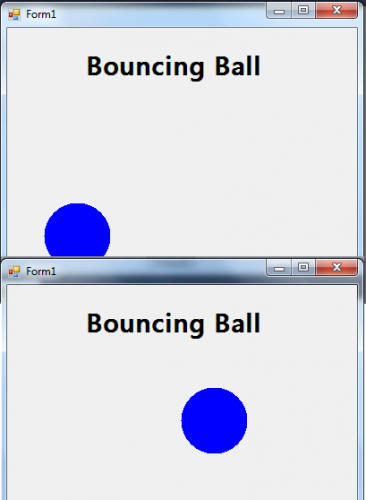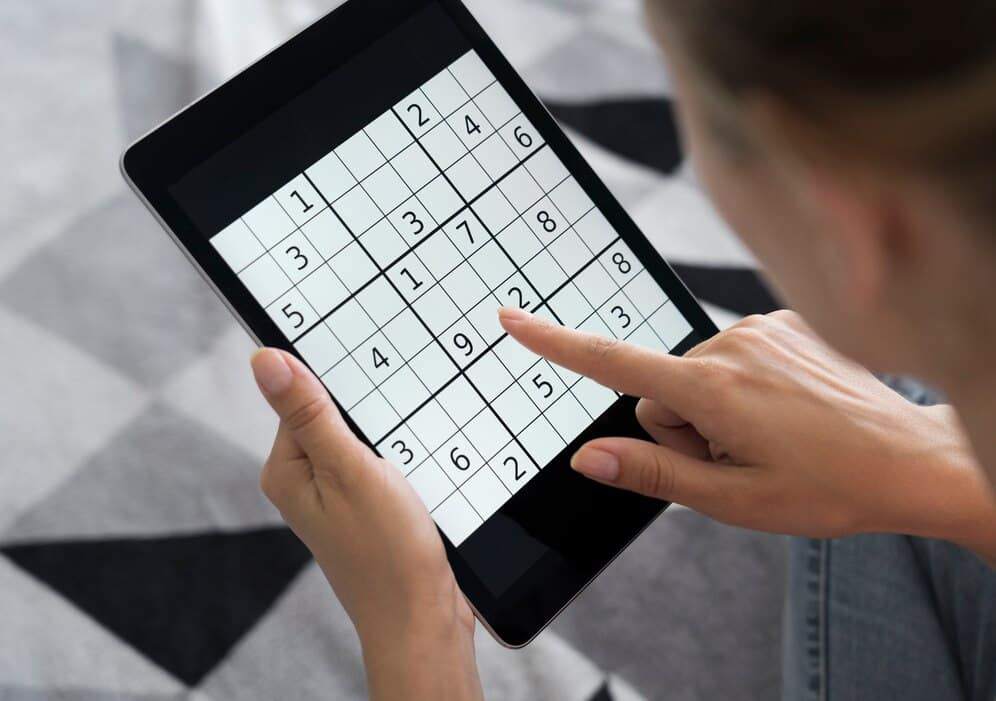Bouncing Ball in C#

# Bouncing Ball In C#

## Outline: Bouncing Ball in C#

Bouncing balls are popular in many games, such as basketball and soccer. Bouncing balls are interesting because they travel in different ways and make different sounds depending on their surfaces. A bouncing ball is an object that moves along a path called a trajectory when it is thrown or dropped and then bounces back to its point of launch, as if repeatedly shooting forward and backward. The trajectory of a bouncing ball is a series of rising and falling segments that form circles, arcs and straight lines. A ball’s bounce can be measured in several ways, such as in terms of its height after bouncing from a flat surface, from an incline or from the air.

In this post, we will illustrate how to develop a program that has an animation of a bouncing ball using the C# programming language.

### Guide: Bouncing Ball in C#

1 – We start by creating a Windows Form Application in C# using the following steps in Microsoft Visual Studio;
– Navigate to File
– Click on New Project
– Select Windows Application

2 – Insert a timer and label it Timer1.
Your interface design should look like the image below;3 – Insert this Global Variable for initiating the ball image and timer in your view code.

```				```
private int ball_Size = 8; //FRACTION OF BALL SIZE
private int move_Size = 4; //FRACTION OF CLIENT AREA
private Bitmap btmp;
private int ball_PositionX;
private int ball_PositionY;
private int ball_MoveX;
private int ball_MoveY;
private int ball_BitmapWidth;
private int ball_BitmapHeight;
private int bitmap_WidthMargin;
private int bitmap_HeightMargin;
```
```

4 – For the Timer1_Tick, the movement of the ball will be initialized by using the below code.

```				```
public void Timer1_Tick(System.Object sender, System.EventArgs e)
{

//DECLARE A VARIABLE TO OBTAIN THE GRAPHICS OBJECT.
Graphics grafx = CreateGraphics();
//DRAW THE BALL IN THE FORM.
grafx.DrawImage(btmp, (int) (ball_PositionX - ball_BitmapWidth / 2), (int) (ball_PositionY - ball_BitmapHeight / 2), ball_BitmapWidth, ball_BitmapHeight);

grafx.Dispose();

//INCREAMENT THE POSITION OF THE BALL BY ITS DISTANCE TO MOVED BOTH X AND Y AXIS.
ball_PositionX += ball_MoveX;
ball_PositionY += ball_MoveY;

//REVERSE THE DIRECTION OF THE BALL WHEN IT HITS TO THE BOUNDARY.
if (ball_PositionX + ball_RadiusX >= ClientSize.Width | ball_PositionX - ball_RadiusX <= 0)
{
ball_MoveX = System.Convert.ToInt32(- ball_MoveX);
Interaction.Beep();
}
//SET THE Y BOUNDARY TO 90 SO THAT IT WILL NOT EXCEED TO THE TITLE OF THE FORM.
if (ball_PositionY + ball_RadiusY >= ClientSize.Height | ball_PositionY - ball_RadiusY <= 90)
{
ball_MoveY = System.Convert.ToInt32(- ball_MoveY);
Interaction.Beep();
}
}

```
```

5 – We will also create a control class to override the OnResize method. This method increases the Resize event which occurs when the control is resized.

```				```
protected override void OnResize(EventArgs ev_arg)
{

Graphics grafx = CreateGraphics();
//ERASE ANY DRAWINGS.
grafx.Clear(BackColor);

//DECLARE A VARIBLE THAT HOLDS THE RADIUS OF THE BALL
//THEN SET THE WIDTH OR THE HIEGHT OF IT TO A FRACTION WHICHEVER IS LESS TO THE CLIENT AREA.
double dbl_Radius = Math.Min(ClientSize.Width / grafx.DpiX, ClientSize.Height / grafx.DpiY) / ball_Size;

//SET THE HIEGHT AND WIDTH OF THE BALL.

grafx.Dispose();
//SET THE DISTANCE THAT THE BALL MOVES INTO 1 PIXEL OR THE BALL SIZE WHICHEVER IS GREATER.
//THIS MEANS THAT THE DISTANCE OF THE BALL MOVES EACH TIME IS PROPORTIONAL TO ITS SIZE,
//WHICH IS ALSO PROPORTIONAL TO THE SIZE OF THE CLIENT AREA.
//THE BALL SLOWS DOWN WHENEVER THE CLIENT AREA IS SHRUNK
//AND THE BALL SPEEDS UP WHEN IT IS INCREASED.

ball_MoveX = (int) (Math.Max(1, ball_RadiusX / move_Size));
ball_MoveY = (int) (Math.Max(1, ball_RadiusY / move_Size));
//THE VALUE OF THE BALL'S MOVEMENT SERVES AS THE MARGIN AROUND THE BALL,
//THAT DETERMINES THE ACTUAL SIZE OF BITMAP ON WHICH THE BALL IS DRAWN.
//THE DISTANCE OF THE BALL MOVES IS EQUAL TO THE SIZE OF THE BITMAP,
//WHICH ALLOWS THE PREVIOUS BALL'S IMAGE TO BE ERASED BEFORE THE NEXT IMAGE IS DRAWN

bitmap_WidthMargin = ball_MoveX;
bitmap_HeightMargin = ball_MoveY;

//TO FIND OUT THE ACTUAL SIZE OF THE BITMAP ON WHICH THE BALL IS DRAWN
//PLUS THE MARGINS TO THE BALL'S DIMENSIONS.
ball_BitmapWidth = 2 * (ball_RadiusX + bitmap_WidthMargin);
ball_BitmapHeight = 2 * (ball_RadiusY + bitmap_HeightMargin);

//CREATE A NEW WIDTH AND HEIGHT OF THE BITMAP.
btmp = new Bitmap(ball_BitmapWidth, ball_BitmapHeight);
//OBTAIN THE GRAFIX OBJECT SHOWN BY THE BITMAP.
grafx = Graphics.FromImage(btmp);
//CLEAR THE EXISTING BALL AND DRAW A NEW BALL.
grafx.Clear(BackColor);
grafx.Dispose();

//RESET THE POSITION OF THE BALL TO THE CENTER OF THE CLIENT AREA.
ball_PositionX = (int) (ClientSize.Width / 2);
ball_PositionY = (int) (ClientSize.Height / 2);

}
```
```

6 – Next, the code below can be used for starting the time in your Form_Load.

```				```
public void Form1_Load(System.Object sender, System.EventArgs e)
{
Timer1.Start();
}
```
```

### Output: Bouncing Ball in C### Recent Posts## Complete School Management System using Python Django

July 8, 2022